Username Password Are you new? Sign up now I’ve forgotten Home :. Downloads :. Articles :. Projects :. Reviews :. Forums :. Arcade :. Klikcast :. GOTW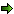Post ReplyPost Oekaki

 Posted By MessagemonkeytheratHero of Time JrRegistered  07/11/2010 Points  1293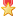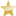20th November, 2010 at 00:07:26 - I was experimenting with some advanced math in movement to make an active go left, up and left, up... and repeat that, but it just stops going up when it reaches the top of the screen, and stops goind left when it reaches the leftmost side of the screen. The coding is like this: Always -set y position of "active" to (tan(sin("sine" active)))+(y position of active) -set x position of "active" to (cos("cosine" active))+(x position of active) -add 3 to sine -add 3 to cosine where sine and cosine are alt values of the active. I think it has something to do with the + x/y position of active , but it doesn't seem like that should make a difference.   If you put a million monkeys at a million keyboards, one of them will eventually write a Java program. The rest of them will write Perl programs.~Matt Esch~Stone GooseRegistered  30/12/2006 Points  87029th November, 2010 at 10:09:08 - The movement you are getting is a result of the way MMF rounds the values. It simply takes the integer part of the number and discards any of the decimal. the cos function is going to be -1<= cos("cosine" active) <= 1, MMF says, for example, if X = 10, Pos X = Pos X + 1 => Pos X = 11 - i.e. you actually move to the right by 1 pixel when cos is equal to exactly 1 Pos X = Pos X + 0.5 => Pos X = 10 - i.e. you don't move anwhere in the x direction when the cos is positve and less than 1 Pos X = Pos X -0.5 => Pos X = 9 - i.e. you move to the left by 1 when the cosine function is negative Pos X = Pos X -1 ==> Pos X = 9 - same as above Now when X = 0 you should end up simply oscillating left and right by 1 pixel because of the following When X = 0 Pos X = Pos X + 1 => Pos X = 1 Pos X = Pos X + 0.5 => Pos X = 1 Pos X = Pos X + 0 ==> Pos X = 1 Pos X = Pos X -0.5 => Pos X = 0 Pos X = Pos X -1 ==> Pos X = -1 Pos X = Pos X - 0.5 ==> Pos X = -1 Pos X = Pos X + 0 ==> Pos X = -1 Pos X = Pos X + 0.5 ==> Pos X = 0 Pos X = Pos X + 1 ==> Pos X = 1 ... etc, note I am just choosing some values of sine to demonstrate, the 0.5 simply reflects the the result of all values 0 < sine < 1 and -0.5 reflects the result of -1 < sine < 0 Similarly, tan( sin( "sine" active ) ) ... sin takes values between -1 and 1 tan( -1 ) = -0.0174551 tan( +1 ) = 0.0174551 So what you see happening is, when sin is negative, the tan function is a small negative number. Adding a small negative number to a positive position, will cause it to move by 1 because MMF says, for example, if Y = 10, Pos Y = Pos Y + 0.0174551 => Pos Y = 10 Pos Y = Pos Y - 0.0174551 => Pos Y = 9 So basically you move up by 1 every loop that the sine function is negative and doesn't move at all in the y direction when the sine function >= 0. It must be the reverse for negative numbers, i.e. taking the integer part is the same as rounding up when your numbers are negative, instead of rounding down when your numbers are positive. So when Y = 0 Pos Y = Pos Y + 0.0174551 => Pos Y = 0 Pos Y = Pos Y - 0.0174551 => Pos Y = 0 So when you get to Y = 0, it stops moving anymore.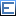Edited by ~Matt Esch~   http://create-games.com/project.asp?id=1875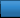Advertisement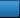Worth A Click